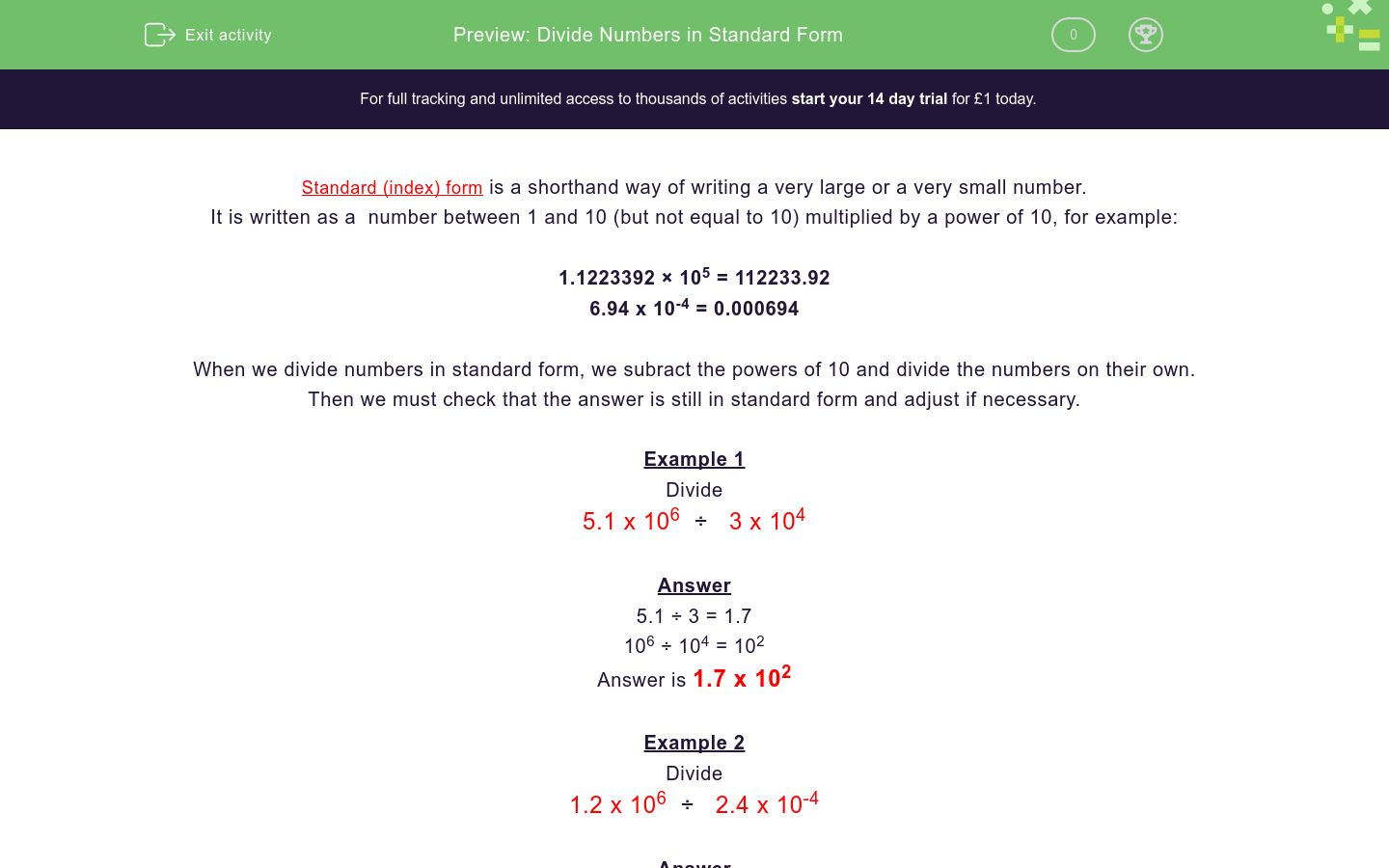# Divide Numbers in Standard Form

In this worksheet, students divide numbers written in standard form.Key stage:  KS 4

Curriculum topic:  Number

Difficulty level:### QUESTION 1 of 10

Standard (index) form is a shorthand way of writing a very large or a very small number.

It is written as a  number between 1 and 10 (but not equal to 10) multiplied by a power of 10, for example:

1.1223392 × 105 = 112233.92

6.94 x 10-4 = 0.000694

When we divide numbers in standard form, we subract the powers of 10 and divide the numbers on their own.

Then we must check that the answer is still in standard form and adjust if necessary.

Example 1

Divide

5.1 x 106  ÷   3 x 104

5.1 ÷ 3 = 1.7

106 ÷ 104 = 102

Answer is 1.7 x 102

Example 2

Divide

1.2 x 106  ÷   2.4 x 10-4

1.2 ÷ 2.4 = 0.5

106 ÷ 10-4 = 1010

Answer is 0.5 x 1010 but this is not in standard form as 0.5 is less than 1.

0.5 = 5 x 10-1

So answer is 5 x 109

Divide

4 x 106  ÷   2 x 104

(The answer must be in standard form)

2 x 101.5

2 x 102

8 x 1010

Divide

3 x 105  ÷   2 x 104

(The answer must be in standard form)

1 x 109

1.5 x 101

1.5 x 109

Divide

7.4 x 108  ÷   3.7 x 104

(The answer must be in standard form)

2 x 104

3.7 x 102

2 x 102

Divide

5 x 108  ÷   4 x 106

(The answer must be in standard form)

1.25 x 1014

20 x 1014

1.25 x 102

Divide

1 x 104  ÷   4 x 101

(The answer must be in standard form)

0.25 x 103

4 x 103

2.5 x 102

Divide

4 x 10-3  ÷   4 x 107

(The answer must be in standard form)

1 x 10-10

8 x 104

1 x 104

Divide

4.2 x 105  ÷   4 x 107

(The answer must be in standard form)

1.05 x 10-2

1.15 x 10-2

0.2 x 1012

Divide

6.3 x 105  ÷   3 x 10-7

(The answer must be in standard form)

2.1 x 10-12

6 x 1013

2.1 x 1012

Divide

1.65 x 105  ÷   3 x 10-7

(The answer must be in standard form)

0.55 x 1012

5.5 x 1011

5.5 x 1013

Divide

1.24 x 10-5  ÷   8 x 107

(The answer must be in standard form)

0.155 x 10-12

1.55 x 10-11

1.55 x 10-13

• Question 1

Divide

4 x 106  ÷   2 x 104

(The answer must be in standard form)

2 x 102
EDDIE SAYS
4 ÷ 2 = 2
106 ÷ 104 = 102
• Question 2

Divide

3 x 105  ÷   2 x 104

(The answer must be in standard form)

1.5 x 101
EDDIE SAYS
3 ÷ 2 = 1.5
105 ÷ 104 = 101
• Question 3

Divide

7.4 x 108  ÷   3.7 x 104

(The answer must be in standard form)

2 x 104
EDDIE SAYS
7.4 ÷ 3.7 = 2
108 ÷ 104 = 104
• Question 4

Divide

5 x 108  ÷   4 x 106

(The answer must be in standard form)

1.25 x 102
EDDIE SAYS
5 ÷ 4 = 1.25
108 ÷ 106 = 102
• Question 5

Divide

1 x 104  ÷   4 x 101

(The answer must be in standard form)

2.5 x 102
EDDIE SAYS
0.25 × 103 is not standard form
• Question 6

Divide

4 x 10-3  ÷   4 x 107

(The answer must be in standard form)

1 x 10-10
EDDIE SAYS
4 ÷ 4 = 1
10-3 ÷ 107 = 10-10
• Question 7

Divide

4.2 x 105  ÷   4 x 107

(The answer must be in standard form)

1.05 x 10-2
EDDIE SAYS
4.2 ÷ 4 = 1.05
105 ÷ 107 = 10-2
• Question 8

Divide

6.3 x 105  ÷   3 x 10-7

(The answer must be in standard form)

2.1 x 1012
EDDIE SAYS
6.3 ÷ 3 = 2.1
105 ÷ 10-7 = 1012
• Question 9

Divide

1.65 x 105  ÷   3 x 10-7

(The answer must be in standard form)

5.5 x 1011
EDDIE SAYS
0.55 × 1012 is not standard form
• Question 10

Divide

1.24 x 10-5  ÷   8 x 107

(The answer must be in standard form)

1.55 x 10-13
EDDIE SAYS
0.155 × 10-12 is not standard form
---- OR ----

Sign up for a £1 trial so you can track and measure your child's progress on this activity.

### What is EdPlace?

We're your National Curriculum aligned online education content provider helping each child succeed in English, maths and science from year 1 to GCSE. With an EdPlace account you’ll be able to track and measure progress, helping each child achieve their best. We build confidence and attainment by personalising each child’s learning at a level that suits them.

Start your £1 trial